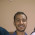Our highest priority is to satisfy the customer through early and continuous delivery of valuable and working software.

## Monday, May 7, 2007

### print_r() function in javascript

function print_r() in JavaScript, identical to php's print_r() function
`function print_r(theObj){   if ( theObj.constructor == Array || theObj.constructor == Object)   {     document.write("<ul>")     for(var p in theObj)     {      if( theObj[p].constructor == Array || theObj[p].constructor == Object)      {        document.write("<li>["+p+"] =>"+typeof(theObj)+"</li>");        document.write("<ul>")        print_r(theObj[p]);        document.write("</ul>")      }      else      {         document.write("<li>["+p+"] =>"+theObj[p]+"</li>");      }     }     document.write("</ul>")   }}`

#### 1 comment:

1.Ya its Working